# Reanalysis of Ratcliff and Rouder (1998) with Diffusion Model and LBA

#### 2019-10-07

This vignette provides the R scripts for a reanalysis of Experiment 1 of Ratcliff and Rouder (1998). In contrast to the original analysis, which used RT bins, we will employ trial-wise maximum likelihood estimation.

The code heavily uses dplyr (vignette), tidyr (vignette), and purrr for data handling and lattice (see ?Lattice) and latticeExtra (specifically as.layer) for plotting. A throrough introduction to the former three packages is provided in R for Data Science by Wickham and Gorlemund, see especially Chapter 25.

# Description of the Experiment

In the experiment, three participants were asked to decide whether the overall brightness of pixel arrays displayed on a computer monitor was “high” or “low”. To this end, the number of white versus black pixels (i.e., the brightness strength) was manipulated in 33 levels from 0% white pixels (level 0) to 100% white pixels (level 32). In addition, instruction manipulated speed and accuracy between blocks. In total, each participant contributed around 4000 trials per instruction condition.

The experiment contained another manipulation, the distribution (or brightness source) from which the pixel array was drawn. One distribution mean was on the “high” brightness side and one distribution mean was on the “low” brightness side. However, as the distributions were unbounded and overlapping, the same strength level could come from either distribution. Participant also received feedback whether or not they had picked the correct distribution (e.g., for the middle strength level 16 probability of belonging to either source was 50%). We do not further consider this manipulation in the following, which seems to be in line with the analysis of Ratcliff and Rouder (1998).

# Descriptive data

As a first step, we load the data and then plot the probability with which each response (i.e., “dark” or “light”) is given as a function of strength and instruction condition. This clearly shows that there is a massive effect of strength on which response is given while at the same time the instruction only seems to have a minor effect and more on the extremes than in the middle.

require(rtdists)
require(dplyr)   # for data manipulations and looping
require(tidyr)   # for data manipulations
require(purrr)   # for data manipulations
require(lattice) # for plotting and corresponding themes
require(latticeExtra)
lattice.options(default.theme = standard.theme(color = FALSE))
lattice.options(default.args = list(as.table = TRUE))
options(digits = 3) # only three decimal digits
require(binom)  # for binomial confidence intervals

data(rr98)
rr98 <- rr98[!rr98$outlier,] #remove outliers # aggregate data for first plot: agg_rr98 <- rr98 %>% group_by(id, instruction, strength) %>% summarise(prop = mean(response == "dark"), mean_rt = mean(rt), median_rt = mean(rt)) %>% ungroup() xyplot(prop ~ strength|id, agg_rr98, group = instruction, type = "b", auto.key = list(lines = TRUE), ylab = "Proportion of 'dark' responses")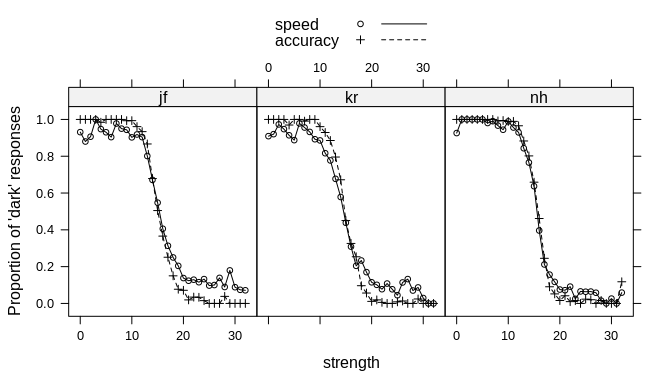Next, we want to get an overview of the response time distributions. For this we look at the response times of the five quantiles (i.e., 0.1, 0.3, 0.5/median, 0.7, 0.9) across the strength manipulations. This time, we also separate the plots by condition as the speed condition resulted in, as expected, vastly shorter response times. These two plots reveal considerable differences between the two instruction conditions. quantiles <- c(0.1, 0.3, 0.5, 0.7, 0.9) ## aggregate data for quantile plot quantiles_rr98 <- rr98 %>% group_by(id, instruction, strength) %>% nest() %>% mutate(quantiles = map(data, ~ as.data.frame(t(quantile(.x$rt, probs = quantiles))))) %>%
unnest(quantiles) %>%
gather("quantile", "rt",10%:90%) %>%
arrange(id, instruction, strength)

xyplot(rt ~ strength|id + instruction, quantiles_rr98, group = quantile, type = "b",
auto.key = list(lines = TRUE), ylab = "RT (in seconds)", subset = instruction == "speed")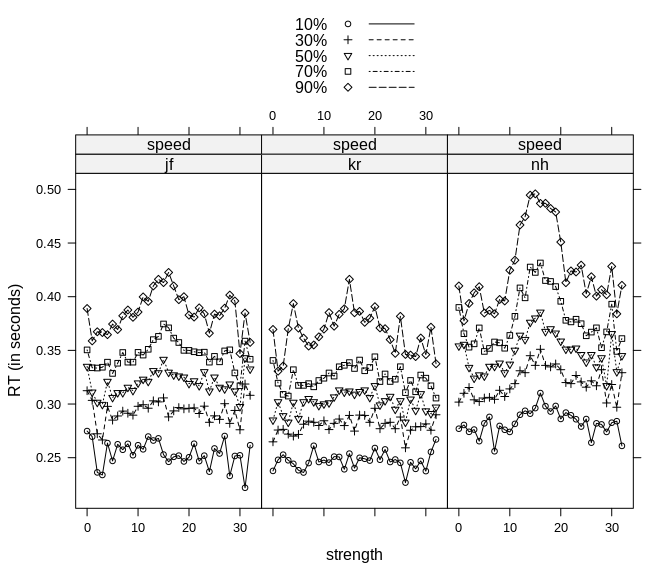xyplot(rt ~ strength|id + instruction, quantiles_rr98, group = quantile, type = "b",
auto.key = FALSE, ylab = "RT (in seconds)", subset = instruction == "accuracy")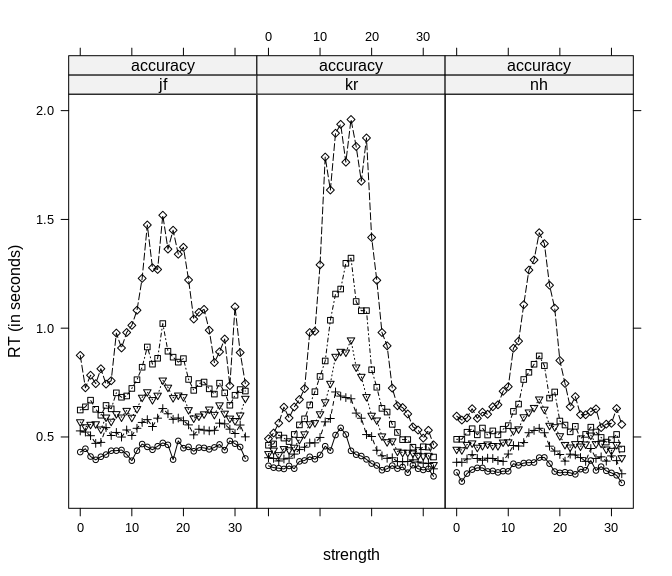In the speed conditions, response times were, as expected, generally fast and there seemed to be hardly any effect of strength. Only for one participant, nh, we can see a small increase in RTs for the higher quantiles for strength values near the middle. In contrast, in the accuracy condition strength has a considerable effect on response times for all participants. Again, this increase was especially strong for the slower responses (i.e., the higher quantiles). For those we see a strong inverse u-shaped effect, symmetrically around the middle – where the probability for each response is 50% – with very high response times for strength values near the middle.

However, as this plot is getting a little bit messy, we now bin the strength levels to remove noise which should provide a clearer overview. For this, we will construct five separate strength bins with approximately equal response behavior and comparable numbers of trials. This is similar to what was done originally by Ratcliff and Rouder (1998). The next table shows the number of trials per participant, bin, and response.

#bins <- c(-0.5, 5.5, 10.5, 13.5, 16.5, 19.5, 25.5, 32.5) # seven bins like RR98
bins <- c(-0.5, 10.5, 13.5, 16.5, 19.5, 32.5)
rr98$strength_bin <- cut(rr98$strength, breaks = bins, include.lowest = TRUE)
levels(rr98$strength_bin) <- as.character(1:7) # aggregate data for response probability plot: agg_rr98_bin <- rr98 %>% group_by(id, instruction, strength_bin) %>% summarise(n1 = n(), dark = sum(response == "dark"), light = sum(response == "light")) %>% ungroup() %>% mutate(prop = map2(dark, n1, ~ binom.confint(.x, .y, methods = "agresti-coull"))) %>% unnest(prop) knitr::kable( rr98 %>% group_by(id, instruction, strength_bin, response) %>% summarise(n = n()) %>% spread(strength_bin, n) ) id instruction response 1 2 3 4 5 jf speed dark 840 467 338 168 146 jf speed light 59 66 284 481 1060 jf accuracy dark 893 496 310 94 30 jf accuracy light 3 44 294 486 1176 kr speed dark 800 379 277 129 102 kr speed light 69 121 350 505 1064 kr accuracy dark 884 469 276 80 9 kr accuracy light 9 71 296 489 1202 nh speed dark 973 552 422 110 81 nh speed light 21 55 276 565 1290 nh accuracy dark 971 535 431 81 23 nh accuracy light 4 32 250 542 1318 We first look again and the response proportions and see more clearly the difference between the strength conditions at the outer bins. xyplot(mean ~ strength_bin|id, agg_rr98_bin, group = instruction, type = "b", auto.key = list(lines = TRUE), ylab = "Proportion of 'dark' responses")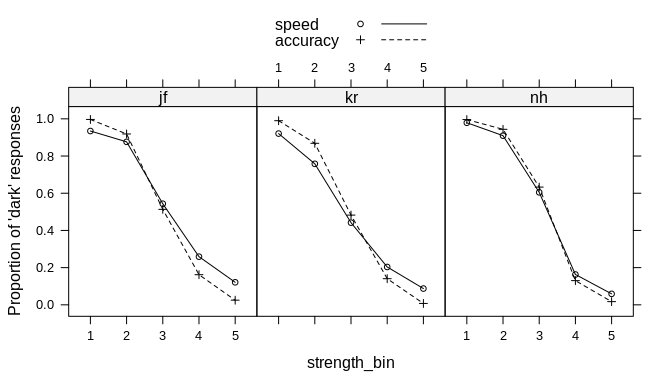Now we also look again at the RT quantiles and see more clearly the symmetrical inverse u-shaped increase in RTs for the middle bins described above. ## aggregate data for quantile plot quantiles_rr98_bin <- rr98 %>% group_by(id, instruction, strength_bin) %>% nest() %>% mutate(quantiles = map(data, ~ as.data.frame(t(quantile(.x$rt, probs = quantiles))))) %>%
unnest(quantiles) %>%
gather("quantile", "rt",10%:90%) %>%
arrange(id, instruction, strength_bin)

xyplot(rt ~ strength_bin|id + instruction, quantiles_rr98_bin, group = quantile, type = "b",
auto.key = list(lines = TRUE), ylab = "RT (in seconds)", subset = instruction == "speed")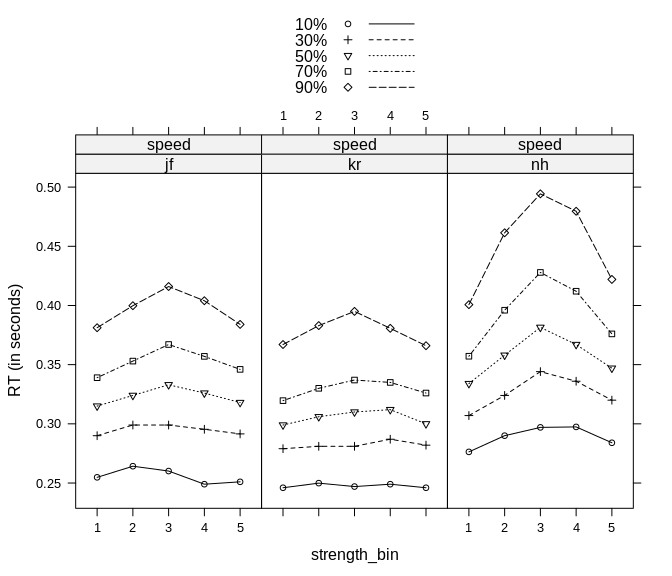xyplot(rt ~ strength_bin|id + instruction, quantiles_rr98_bin, group = quantile, type = "b",
auto.key = FALSE, ylab = "RT (in seconds)", subset = instruction == "accuracy")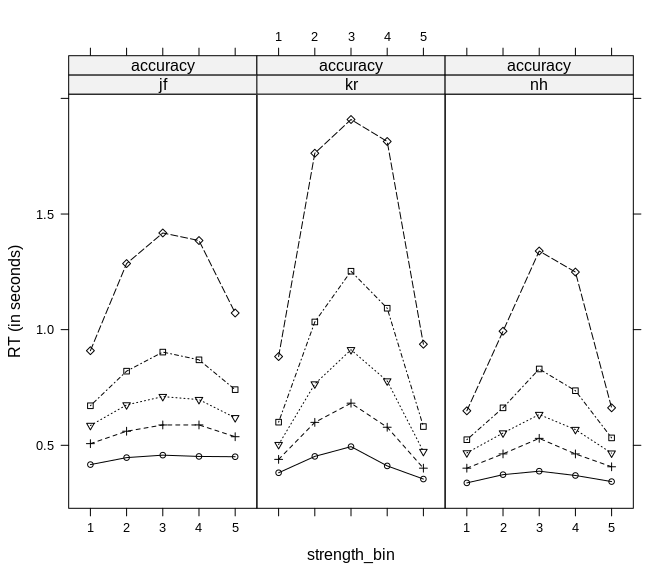With this clear pattern we now take a look at the RT distributions separately for both responses to see if they are simply mirror images of each other or not. For this, we overlay the two RT quantile plots for all trials in which the responses was “dark” in black (there are more “dark” pixels for the bins on the left side of the plot) with the same plot in which the responses was “light” in grey (there are more “light” pixels for the bins on the right side of the plot).

agg2_rr98_response <- rr98  %>%
group_by(id, instruction, strength_bin, response) %>%
nest() %>%
mutate(quantiles = map(data, ~ as.data.frame(t(quantile(.x$rt, probs = quantiles))))) %>% unnest(quantiles) %>% gather("quantile", "rt",10%:90%) %>% arrange(id, instruction, response, strength_bin) p1 <- xyplot(rt ~ strength_bin|id, agg2_rr98_response, group = quantile, type = "b", auto.key = list(lines = TRUE), ylab = "RT (in seconds)", subset = instruction == "speed" & response == "dark", layout = c(3,1)) p2 <- xyplot(rt ~ strength_bin|id, agg2_rr98_response, group = quantile, type = "b", auto.key = list(lines = TRUE), ylab = "RT (in seconds)", subset = instruction == "speed" & response == "light", col = "grey") p1 + as.layer(p2)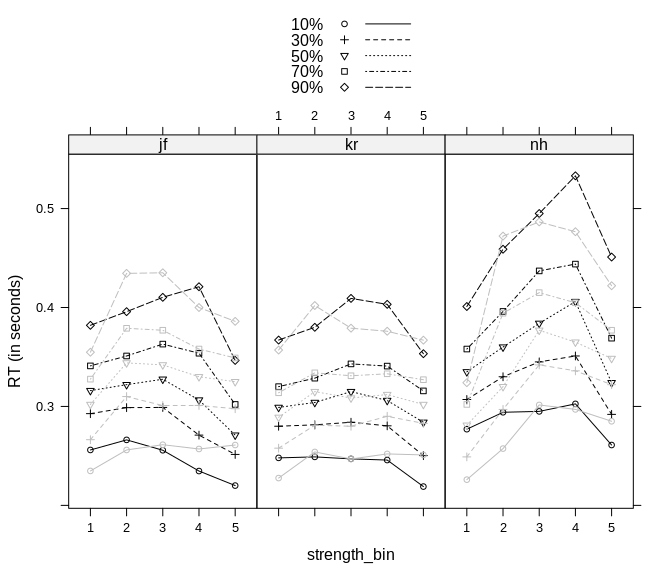p1 <- xyplot(rt ~ strength_bin|id, agg2_rr98_response, group = quantile, type = "b", auto.key = list(lines = TRUE), ylab = "RT (in seconds)", subset = instruction == "accuracy" & response == "dark", layout = c(3,1)) p2 <- xyplot(rt ~ strength_bin|id, agg2_rr98_response, group = quantile, type = "b", auto.key = list(lines = TRUE), ylab = "RT (in seconds)", subset = instruction == "accuracy" & response == "light", col = "grey") p1 + as.layer(p2)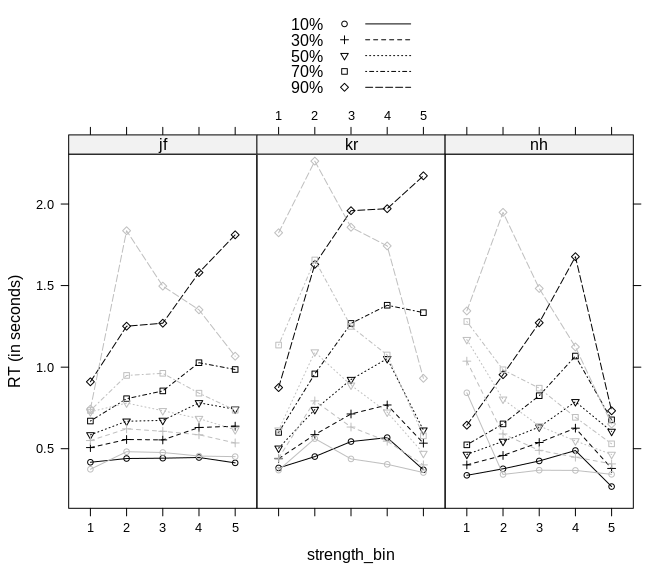These two plots reveal an interesting pattern. In the speed condition (upper plot), we particularly see fast “errors” (i.e., responses to “dark” when there are more light pixels or the other way round). When “dark” is the more likely response (i.e. on the left side) the “light” responses in grey are faster and this is especially true for the lower quantiles. The opposite pattern seems to hold on the opposite side where “dark” responses in black are faster than “light” responses in grey. At intermediate bins the difference seems to be rather at the higher quantiles. This is particularly noticeable for participant kr for which for there seem to be slow “light”-“errors” just to the left to the middle bin and slow “right”-“errors” just to the right of the middle bin. For the accuracy condition in the lower plot the pattern is noticeably different. First of all, there are only very few or no “error” responses in the extreme bins. Consequently, there does not seem to be any evidence for fast errors at the extremes (and also not at intermediate strength levels). However, we here more clearly see the slow errors at the intermediate bins. When “dark” is somewhat more probably (i.e., to the left of the middle) “light” responses are noticeably slower than “dark” responses. The same holds for “dark” responses if “light” is more probable. Importantly, this shows that the symmetrical inverse u-shaped increase for the middle bins described above is actually a consequence of a mixture of slow “errors”, two asymmetric increases for the two different responses. # Diffusion Model Analysis We will follow Ratcliff and Rouder (1998) and analyze the data with the diffusion model. For this, we will fit a separate model to each participant and instruction condition. To do so, we will first create a new data set we will use for fitting. This data set will be nested with one row for each combinations of the variables: d_nested <- rr98 %>% group_by(id, instruction) %>% # we loop across both, id and instruction nest() d_nested ## # A tibble: 6 x 3 ## # Groups: id, instruction  ## id instruction data ## <fct> <fct> <list<df[,11]>> ## 1 jf accuracy [3,826 × 11] ## 2 jf speed [3,909 × 11] ## 3 kr accuracy [3,785 × 11] ## 4 kr speed [3,796 × 11] ## 5 nh speed [4,345 × 11] ## 6 nh accuracy [4,187 × 11] Like Ratcliff and Rouder we will fit the data to the strength bins instead of the full strength manipulation. We fit basically the full diffusion model (with the exception of $$s_{t0}$$) to each instruction condition which results in 10 parameters per participant and instruction condition: • 5 drift rates $$v$$ (i.e., one per strength bin) • 1 boundary separation $$a$$ • 1 non-decision time $$t_0$$ • 1 drift rate variability $$s_v$$ • 1 start point $$z$$ (for ease in interpretation we parameterize this as the relative start point so that values different from 0.5 indicate a bias towards one of the responses) • 1 start point variability $$s_z$$ Like in Ratcliff and Rouder (1998), the two response boundaries are the two response options “dark” and “light”. To estimate the model we diverge from Ratcliff and Rouder and employ trial wise maximum likelihood estimation (i.e., no binning of responses). For this, we simply need to have a wrapper function which returns us the negative summed log-likelihood of the data (i.e., RTs and corresponding responses) given a set of parameters. We need the negativ sum because most optimization function minimize whereas we want to obtain the maximum likelihood value. The following function for which we simply loop across drift rates will do so: # objective function for diffusion with 1 a. loops over drift to assign drift rates to strength objective_diffusion_separate <- function(pars, rt, response, drift, ...) { non_v_pars <- grep("^v", names(pars), invert = TRUE, value = TRUE) base_par <- length(non_v_pars) # number of non-drift parameters densities <- vector("numeric", length(rt)) for (i in seq_along(levels(drift))) { densities[drift == levels(drift)[i]] <- ddiffusion(rt[drift == levels(drift)[i]], response=response[drift == levels(drift)[i]], a=pars["a"], t0=pars["t0"], sv=pars["sv"], sz=if ("sz" %in% non_v_pars) pars["sz"] else 0.1, z=if ("z" %in% non_v_pars) pars["z"]*pars["a"] else 0.5*pars["a"], st0=if ("st0" %in% non_v_pars) pars["st0"] else 0, v=pars[base_par+i]) } if (any(densities == 0)) return(1e6) return(-sum(log(densities))) } Note that the function is written in such a way that we could easily fix certain parameters without the necessity to change it (using if-then on the parameters names passed via pars). Additionally, we also need a function that generates a set of random starting values. And, as any random set of starting values may be impossible, another wrapper function that generates starting values until a set of valid starting values is found and then passes those to the optimization routine. As optimization routine we will be using nlminb. These functions are given next and are specified in a way that they will be usable for other model variants for this data (e.g., fixing parameters). # function that creates random start values, also get_start <- function(base_par, n_drift = 5) { start1 <- c( a = runif(1, 0.5, 3), a_1 = runif(1, 0.5, 3), a_2 = runif(1, 0.5, 3), t0 = runif(1, 0, 0.5), z = runif(1, 0.4, 0.6), sz = runif(1, 0, 0.5), sv = runif(1, 0, 0.5), st0 = runif(1, 0, 0.5), d = rnorm(1, 0, 0.05) ) start2 <- sort(rnorm(n_drift), decreasing = FALSE) names(start2) <- paste0("v_", seq_len(n_drift)) c(start1[base_par], start2) } # function that tries different random start values until it works: ensure_fit <- function(data, start_function, objective_function, base_pars, n_drift = 5, n_fits = 1, lower = c(rep(0, length(base_pars)), -Inf, rep(-Inf,length(start_function(base_pars))-length(base_pars)))) { best_fit <- list(objective = 1e+06) for (i in seq_len(n_fits)) { start_ll <- 1e+06 #browser() while(start_ll == 1e+06) { start <- start_function(base_pars, n_drift=n_drift) start_ll <- objective_function(start, rt = data$rt, response = data$response_num, drift = factor(data$strength_bin, seq_len(n_drift)),
instruction = data$instruction) } cat("\nstart fitting.\n") # just for information to see if it is stuck fit <- nlminb(start, objective_function, rt = data$rt, response = data$response_num, drift = factor(data$strength_bin, seq_len(n_drift)),
instruction = data$instruction, lower = lower) if (fit$objective < best_fit$objective) best_fit <- fit } out <- as.data.frame(t(unlist(best_fit[1:3]))) colnames(out) <- sub("par.", "", colnames(out)) out } With these functions in place, we now simply need to loop over participants and items to obtain the fit. The simplest way is perhaps to use the combination of purrr:map and dplyr::mutate as shown here: fit_diffusion <- d_nested %>% mutate(fit = map(data, ~ensure_fit(data = ., start_function = get_start, objective_function = objective_diffusion_separate, base_pars = c("a", "t0", "sv", "sz", "z")))) %>% unnest(fit) On Unix-like systems (i.e., Linux and Mac) we can easily use the inbuild multicore functionality using parallel::mclapply to distribute fitting of the different parts across different cores: require(parallel) fit_diffusion <- d_nested fit_diffusion$fit <-
mclapply(d_nested$data, function(x) ensure_fit(data = x, start_function = get_start, objective_function = objective_diffusion_separate, base_pars = c("a", "t0", "sv", "sz", "z")), mc.cores = 2) fit_diffusion <- unnest(fit_diffusion, fit) The following table gives the parameter values, the negative summed log-likelihoods (i.e., objective, where smaller is better), and the convergence code of the optimization algorithm (where 0 indicates no problem) obtained from this fit: fit_diffusion$data <- NULL
if (!("st0" %in% colnames(fit_diffusion))) fit_diffusion$st0 <- 0 if (!("z" %in% colnames(fit_diffusion))) fit_diffusion$z <- 0.5
if (!("sz" %in% colnames(fit_diffusion))) fit_diffusion$sz <- 0.1 knitr::kable(fit_diffusion) id instruction a t0 sv sz z v_1 v_2 v_3 v_4 v_5 objective convergence st0 jf accuracy 1.979 0.221 0.496 0.208 0.488 -2.39 -1.52 -0.018 1.22 2.07 1301 0 0 jf speed 0.877 0.195 0.000 0.222 0.468 -2.92 -2.28 -0.075 1.83 2.94 -3200 0 0 kr accuracy 2.196 0.212 1.077 0.250 0.549 -3.55 -1.60 -0.070 1.23 3.12 1228 0 0 kr speed 0.819 0.196 0.196 0.216 0.475 -2.99 -1.66 0.550 2.18 3.25 -3489 0 0 nh speed 1.130 0.196 0.000 0.188 0.524 -4.03 -2.83 -0.746 1.87 3.02 -3318 0 0 nh accuracy 1.843 0.227 1.001 0.547 0.519 -3.80 -2.35 -0.620 1.67 3.38 -245 0 0 We can see from these values that there is a large effect of instruction on $$a$$. However, instruction also has effects on other parameters: • $$t_0$$ is consistently larger in the accuracy compared to the speed condition, although this effect is small. • $$s_v$$ is estimated at 0 or very low in the speed condition, but 0.5 or 1 in the accuracy condition. This is consistent with the absence of slow “errors” in the speed condition. • $$s_z$$ is consistently larger in the speed conditions consistent with the presence or more fast “errors” in the speed than in the accuracy condition. • $$v$$ appears to be more extreme (i.e., smaller for $$v_1$$ and $$v_2$$ and larger for $$v_4$$ and $$v_5$$) in the speed compared to the accuracy condition. ## Graphical Model Fit ### Predicted Response Rates To evaluate the fits graphically we first compare the actual response rates for the two responses with the predicted responses rates. The grey lines and points show the observed data and the error bars are binomial confidence intervals. The black lines and points show the predicted response rates. # get predicted response proportions pars_separate_l <- fit_diffusion %>% gather("strength_bin", "v", starts_with("v")) pars_separate_l$strength_bin <- factor(substr(pars_separate_l$strength_bin, 3,3), levels = as.character(seq_len(length(bins)-1))) #pars_separate_l <- inner_join(pars_separate_l, agg_rr98_bin) pars_separate_l <- pars_separate_l %>% group_by(id, instruction, strength_bin) %>% mutate(resp_prop = pdiffusion(rt=Inf, response="lower", a=a, v=v, t0=t0, sz = sz, z=a*z, sv=sv, st0=st0)) p1 <- xyplot(mean ~ strength_bin|id + instruction, agg_rr98_bin, type = "b", auto.key = list(lines = TRUE), ylab = "Proportion of 'dark' responses", col = "grey") p2 <- segplot(strength_bin ~ upper+lower|id + instruction, agg_rr98_bin, auto.key = list(lines = TRUE), ylab = "Proportion of 'dark' responses", col = "grey", horizontal = FALSE, segments.fun = panel.arrows, draw.bands = FALSE, angle = 90, length = 0.05, ends = "both") p3 <- xyplot(resp_prop ~ strength_bin|id + instruction, pars_separate_l, type = "b", auto.key = list(lines = TRUE), ylab = "Proportion of 'dark' responses", col = "black") p2 + as.layer(p1) + as.layer(p3)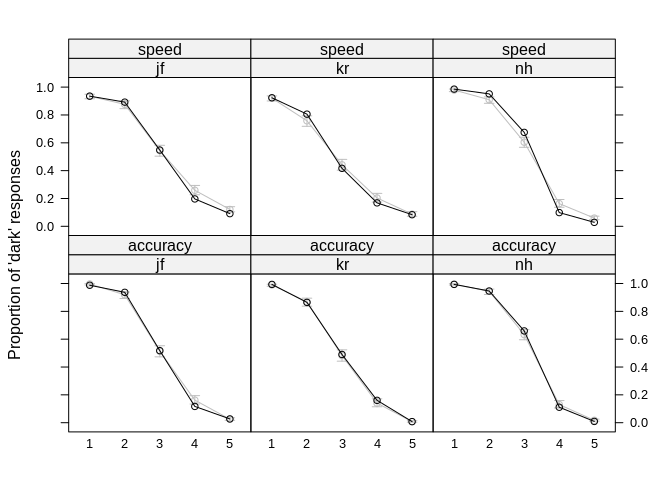This figure show that overall the model can predict the actual response rates very accurately. There are only a few minor deviations. ### Predicted Median RTs Next we compare the central tendency of the RTs with the prediction. For this we evaluate the CDF at the quantiles of the predicted response proportions. Again, the data is shown in grey (and error bars show the standard errors of the median) and the predicted RTs in black. We first show the predictions for the speed condition, separated by response. # get predicted quantiles (uses predicted response proportions) separate_pred_dark <- pars_separate_l %>% do(as.data.frame(t( qdiffusion(quantiles*.$resp_prop, response="lower",
a=.$a, v=.$v, t0=.$t0, sz = .$sz, z = .$z*.$a, sv=.$sv, st0=.$st0)))) %>%
ungroup() %>% gather("quantiles", "dark", V1:V5)
separate_pred_light <- pars_separate_l %>% do(as.data.frame(t(
mclapply(d_nested$data, function(x) ensure_fit(data = x, start_function = get_start_lba, objective_function = objective_lba_separate, base_pars = c("a_1", "a_2", "t0", "b", "sv"), lower = c(rep(-Inf, 5), rep(0, 5)), n_drift = 5, n_fits = 10), mc.cores = 2) fit_lba <- unnest(fit_lba, fit) The following table gives the parameters and the negative summed log-likelihoods obtained from the LBA fit (with $$b$$ already correctly transformed by the maximum $$A$$). Note that some of the parameters might differ slightly for for id = kr and instruction = accuracy although the value of the objective function is identical to the reported one. This suggests that the likelihood surface is either quite shallow near the MLE or there are at least two peaks in the likelihood surface with a similar maximum. The fact that the convergence code for this data set is 1 instead of 0 also suggests some problems in finding the gloval optimum. In any case, running the optimization multiple times and comparing the results should reveal such problems. knitr::kable(fit_lba) id instruction v_1 v_2 v_3 v_4 v_5 a_1 a_2 t0 b sv objective convergence jf accuracy 0.964 0.793 0.508 0.259 0.096 0.359 0.331 0.049 0.327 0.293 1171 0 jf speed 0.586 0.565 0.494 0.439 0.406 0.084 0.067 0.014 0.133 0.100 -3855 0 kr accuracy 3.580 2.101 0.526 -0.812 -2.413 0.596 0.885 0.107 0.863 1.383 1358 1 kr speed 0.619 0.563 0.471 0.411 0.368 0.075 0.066 0.083 0.096 0.136 -4033 0 nh speed 0.678 0.613 0.529 0.425 0.378 0.065 0.076 0.038 0.155 0.123 -4235 0 nh accuracy 1.448 1.100 0.644 0.022 -0.381 0.387 0.405 0.144 0.238 0.456 -227 0 The negtaive log-likelihood (column objective) shows that the LBA provides a better account for four of the six data sets (because it is the negative log-likelihood, smaller is better). The diffusion model only provides a better account for the kr and nh accuracy conditions. In terms of the parameter estimates we see a pattern similar as the one for the diffusion model: • Instruction shows the expected effect on $$b$$. • Instruction also shows an effect on $$A$$, which is also larger in the accuracy compared to the speed condition. • Instruction seems to have a small effect on $$t_0$$ in the same direction. • Instruction also affected $$s_v$$ in the same direction. • Instruction also affected $$v$$ which seemed to be more extreme in the accuracy compared to the speed condition. ## Graphical Model Fit The fact that the LBA provides a slightly better fit is also visible in the graphical fit assessment. ### Predicted Response Rates We again first consider the predicted response rates (in black) and they are also highly accurate. # get predicted response proportions lba_pars_separate_l <- fit_lba %>% gather("strength_bin", "v", starts_with("v")) lba_pars_separate_l$strength_bin <- factor(substr(lba_pars_separate_l$strength_bin, 3,3), levels = as.character(seq_len(length(bins)-1))) #pars_separate_l <- inner_join(pars_separate_l, agg_rr98_bin) lba_pars_separate_l <- lba_pars_separate_l %>% group_by(id, instruction, strength_bin) %>% mutate(resp_prop = pLBA(rt=Inf, response=1, A=list(a_1, a_2), sd_v=sv, mean_v=c(v, 1-v), t0=t0, b=max(a_1, a_2)+b, silent=TRUE)) p1 <- xyplot(mean ~ strength_bin|id + instruction, agg_rr98_bin, type = "b", auto.key = list(lines = TRUE), ylab = "Proportion of 'dark' responses", col = "grey") p2 <- segplot(strength_bin ~ upper+lower|id + instruction, agg_rr98_bin, auto.key = list(lines = TRUE), ylab = "Proportion of 'dark' responses", col = "grey", horizontal = FALSE, segments.fun = panel.arrows, draw.bands = FALSE, angle = 90, length = 0.05, ends = "both") p3 <- xyplot(resp_prop ~ strength_bin|id + instruction, lba_pars_separate_l, type = "b", auto.key = list(lines = TRUE), ylab = "Proportion of 'dark' responses", col = "black") p2 + as.layer(p1) + as.layer(p3)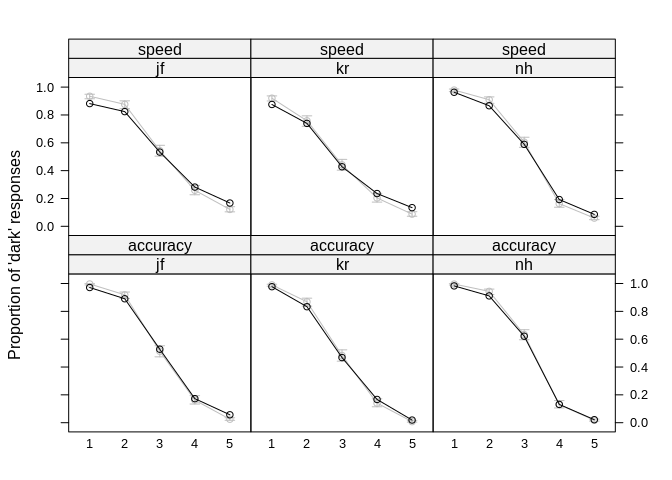### Predicted Median RTs Again, the data is shown in grey (and error bars show the standard errors of the median) and the predicted RTs in black. We first show the predictions for the speed condition, separated by response. # get predicted quantiles (uses predicted response proportions) lba_separate_pred_dark <- lba_pars_separate_l %>% do(as.data.frame(t( qLBA(quantiles*.$resp_prop, response=1, A=list(.$a_1, .$a_2), sd_v=.$sv, mean_v=c(.$v, 1-.$v), t0=.$t0, b=max(.$a_1, .$a_2)+.$b, silent=TRUE)))) %>% ungroup() %>% gather("quantiles", "dark", V1:V5) lba_separate_pred_light <- lba_pars_separate_l %>% do(as.data.frame(t( qLBA(quantiles*(1-.$resp_prop), response=2, A=list(.$a_1, .$a_2), sd_v=.$sv, mean_v=c(.$v, 1-.$v), t0=.$t0, b=max(.$a_1, .$a_2)+.$b, silent=TRUE)))) %>% ungroup() %>% gather("quantiles", "light", V1:V5) #separate_pred_light %>% filter(is.na(light)) lba_separate_pred <- inner_join(lba_separate_pred_dark, lba_separate_pred_light) lba_separate_pred$quantiles <- factor(lba_separate_pred$quantiles, levels = c("V5", "V4", "V3", "V2", "V1"), labels = c("90%", "70%", "50%", "30%", "10%")) lba_separate_pred <- lba_separate_pred %>% gather("response", "rt", dark, light) p1 <- xyplot(rt ~ strength_bin|id+response, agg2_rr98_response, type = "b", auto.key = list(lines = TRUE), ylab = "RT (in seconds)", subset = instruction == "speed" & quantile == "50%", layout = c(3,2), col = "grey") p1e <- segplot(strength_bin ~ upper+lower|id+response, agg2_rr98_response, auto.key = list(lines = TRUE), ylab = "Proportion of 'dark' responses", col = "grey", horizontal = FALSE, segments.fun = panel.arrows, draw.bands = FALSE, angle = 90, length = 0.05, ends = "both", subset = instruction == "speed" & quantile == "50%", layout = c(3,2)) p2 <- xyplot(rt ~ strength_bin|id + response, lba_separate_pred, type = "b", auto.key = list(lines = TRUE), ylab = "RT (in seconds)", subset = instruction == "speed" & quantiles == "50%", scales = list(y = list(limits = c(0.25, 0.5)))) p2 + as.layer(p1) + as.layer(p1e)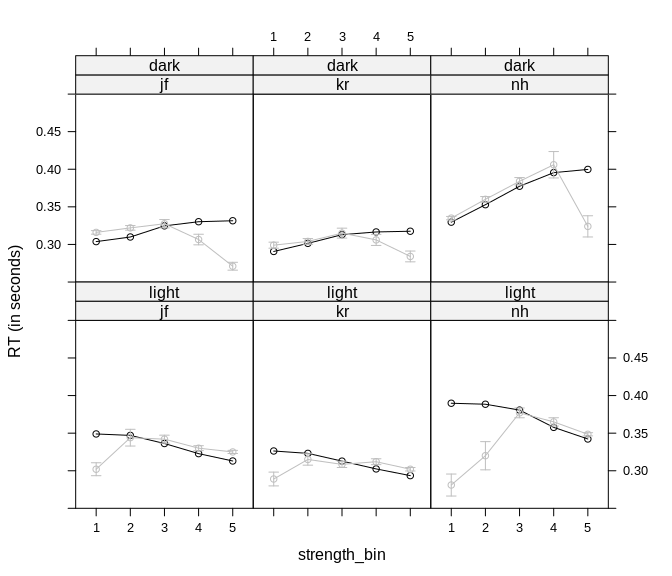Next shows the same plot for the accuracy condition. p1 <- xyplot(rt ~ strength_bin|id+response, agg2_rr98_response, type = "b", auto.key = list(lines = TRUE), ylab = "RT (in seconds)", subset = instruction == "accuracy" & quantile == "50%", layout = c(3,2), col = "grey") p1e <- segplot(strength_bin ~ upper+lower|id+response, agg2_rr98_response, auto.key = list(lines = TRUE), ylab = "Proportion of 'dark' responses", col = "grey", horizontal = FALSE, segments.fun = panel.arrows, draw.bands = FALSE, angle = 90, length = 0.05, ends = "both", subset = instruction == "accuracy" & quantile == "50%", layout = c(3,2)) p2 <- xyplot(rt ~ strength_bin|id + response, lba_separate_pred, type = "b", auto.key = list(lines = TRUE), ylab = "RT (in seconds)", subset = instruction == "accuracy" & quantiles == "50%", scales = list(y = list(limits = c(0.2, 1.5)))) p2 + as.layer(p1) + as.layer(p1e)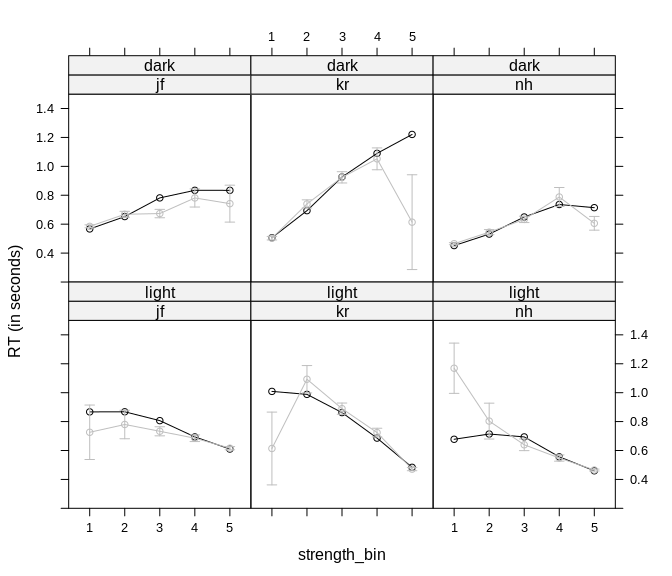Overall the LBA is able to describe the central tendency relatively well. The only considerable misfit is evident at the extreme bins with few responses (i.e., large error bars). ### All quantiles Next, we investigate the full RT distribution by comparing observed and predicted quantiles. The observed quantiles are again displayed in grey and the predictions in black. The first plot shows the speed condition separated by response. p1 <- xyplot(rt ~ strength_bin|id+response, agg2_rr98_response, group = quantile, type = "b", auto.key = list(lines = TRUE), ylab = "RT (in seconds)", subset = instruction == "speed", layout = c(3,2), col = "grey") p1e <- segplot(strength_bin ~ upper+lower|id+response, agg2_rr98_response, auto.key = list(lines = TRUE), ylab = "Proportion of 'dark' responses", col = "grey", horizontal = FALSE, segments.fun = panel.arrows, draw.bands = FALSE, angle = 90, length = 0.05, ends = "both", subset = instruction == "speed") p2 <- xyplot(rt ~ strength_bin|id + response, lba_separate_pred, group = quantiles, type = "b", auto.key = list(lines = TRUE), ylab = "RT (in seconds)", subset = instruction == "speed", scales = list(y = list(limits = c(0.2, 0.6)))) p2 + as.layer(p1) + as.layer(p1e)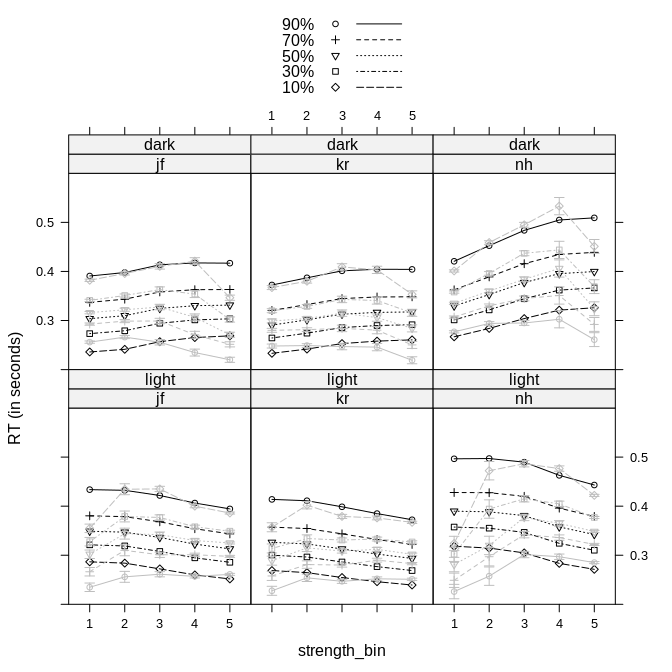The next plot shows the accuracy condition separated by response. p1 <- xyplot(rt ~ strength_bin|id+response, agg2_rr98_response, group = quantile, type = "b", auto.key = list(lines = TRUE), ylab = "RT (in seconds)", subset = instruction == "accuracy", layout = c(3,2), col = "grey") p1e <- segplot(strength_bin ~ upper+lower|id+response, agg2_rr98_response, auto.key = list(lines = TRUE), ylab = "Proportion of 'dark' responses", col = "grey", horizontal = FALSE, segments.fun = panel.arrows, draw.bands = FALSE, angle = 90, length = 0.05, ends = "both", subset = instruction == "accuracy") p2 <- xyplot(rt ~ strength_bin|id + response, lba_separate_pred, group = quantiles, type = "b", auto.key = list(lines = TRUE), ylab = "RT (in seconds)", subset = instruction == "accuracy", scales = list(y = list(limits = c(0.1, 3.3)))) p2 + as.layer(p1) + as.layer(p1e)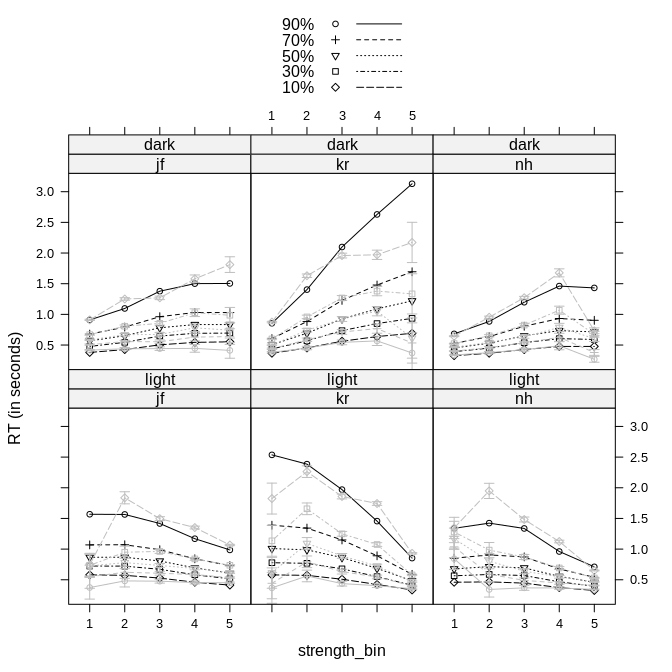These two plots show not only the central tendency, but the other quantiles are quite well described. # Comparing Model Fit Finally, we graphically compare the fit of the two models. Here we can see that while the LBA seems to provide a slightly better fit (as indicated by the lower negative log-likelihoods), the diffusion model seems to somewhat better recover some of the trends in the data. ## Predicted Response Rates We again first consider the predicted response rates (in black) for the two models. key <- simpleKey(text = c("data", "LBA", "Diffusion"), lines = TRUE) key$lines$col <- c("grey", "black", "black") key$lines$lty <- c(1, 1, 2) key$points$col <- c("grey", "black", "black") key$points\$pch <- c(1, 0, 4)

p1 <- xyplot(mean ~ strength_bin|id + instruction, agg_rr98_bin, type = "b", auto.key =
list(lines = TRUE), ylab = "Proportion of 'dark' responses", col = "grey")
p2 <- segplot(strength_bin ~ upper+lower|id + instruction, agg_rr98_bin,
auto.key = list(lines = TRUE), ylab = "Proportion of 'dark' responses",
col = "grey", horizontal = FALSE, segments.fun = panel.arrows,
draw.bands = FALSE, angle = 90, length = 0.05, ends = "both")
p3 <- xyplot(resp_prop ~ strength_bin|id + instruction, lba_pars_separate_l, type = "b",
auto.key = list(lines = TRUE), ylab = "Proportion of 'dark' responses",
col = "black", pch = 0)
p4 <- xyplot(resp_prop ~ strength_bin|id + instruction, pars_separate_l, type = "b",
auto.key = list(lines = TRUE), ylab = "Proportion of 'dark' responses",
col = "black", lty = 2, key = key, pch=4)
p4 + as.layer(p2) + as.layer(p1) + as.layer(p3)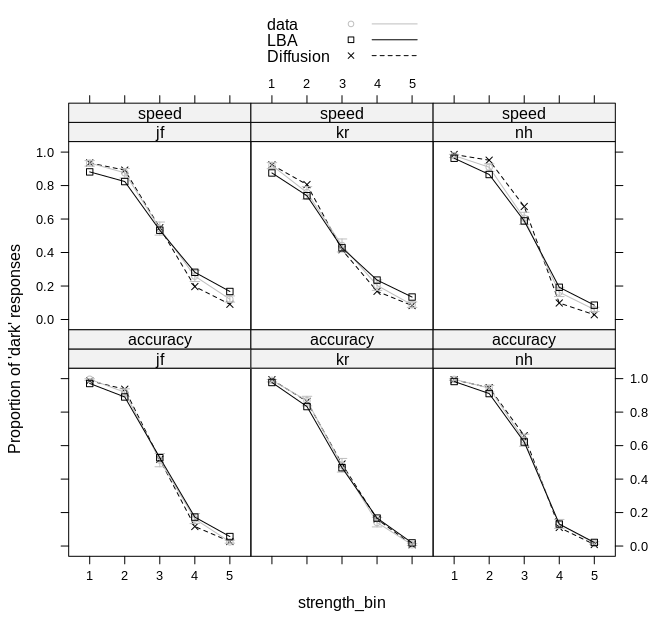When comparing the fit of the two models like this, it shows that both models make very similar predictions for the predicted response rates. It is difficult to see huge differences between the models.

## Predicted Median RTs

Next, we also compare the two accounts for the median. As before, data is shown in grey and we begin with a plot for the speed condition, separated by response.

p1 <- xyplot(rt ~ strength_bin|id+response, agg2_rr98_response, type = "b",
auto.key = list(lines = TRUE), ylab = "RT (in seconds)",
subset = instruction == "speed" & quantile == "50%",
layout = c(3,2), col = "grey")
p1e <- segplot(strength_bin ~ upper+lower|id+response, agg2_rr98_response,
auto.key = list(lines = TRUE), ylab = "Proportion of 'dark' responses",
col = "grey", horizontal = FALSE, segments.fun = panel.arrows,
draw.bands = FALSE, angle = 90, length = 0.05, ends = "both",
subset = instruction == "speed" & quantile == "50%", layout = c(3,2))
p2 <- xyplot(rt ~ strength_bin|id + response, lba_separate_pred, type = "b",
auto.key = list(lines = TRUE), ylab = "RT (in seconds)",
subset = instruction == "speed" & quantiles == "50%",
scales = list(y = list(limits = c(0.25, 0.5))), pch = 0)
p3 <- xyplot(rt ~ strength_bin|id + response, separate_pred, type = "b",
auto.key = list(lines = TRUE), ylab = "RT (in seconds)",
subset = instruction == "speed" & quantiles == "50%",
scales = list(y = list(limits = c(0.25, 0.5))),
col = "black", lty = 2, key = key, pch=4)

p3 + as.layer(p2) + as.layer(p1) + as.layer(p1e)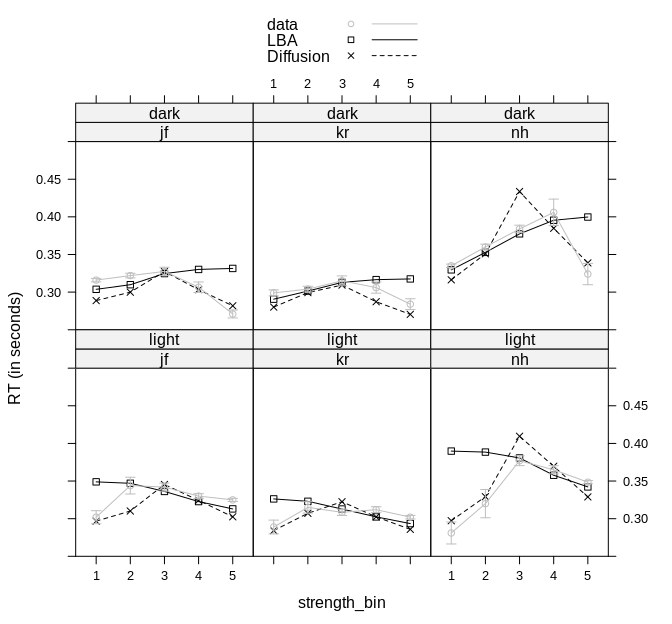This plot suggests that the diffusion model is better able to predict changes in the median RTs in the speed condition across strength bins than the LBA. While this leads to obvious misfit in certain cases (dark responses for nh) it appears to provide a generally better recovery of the patterns in the data.

Next shows the same plot for the accuracy condition.

p1 <- xyplot(rt ~ strength_bin|id+response, agg2_rr98_response, type = "b",
auto.key = list(lines = TRUE), ylab = "RT (in seconds)",
subset = instruction == "accuracy" & quantile == "50%",
layout = c(3,2), col = "grey")
p1e <- segplot(strength_bin ~ upper+lower|id+response, agg2_rr98_response,
auto.key = list(lines = TRUE), ylab = "Proportion of 'dark' responses",
col = "grey", horizontal = FALSE, segments.fun = panel.arrows,
draw.bands = FALSE, angle = 90, length = 0.05, ends = "both",
subset = instruction == "accuracy" & quantile == "50%", layout = c(3,2))
p2 <- xyplot(rt ~ strength_bin|id + response, lba_separate_pred, type = "b",
auto.key = list(lines = TRUE), ylab = "RT (in seconds)",
subset = instruction == "accuracy" & quantiles == "50%",
pch = 0)
p3 <- xyplot(rt ~ strength_bin|id + response, separate_pred, type = "b",
auto.key = list(lines = TRUE), ylab = "RT (in seconds)",
subset = instruction == "accuracy" & quantiles == "50%",
scales = list(y = list(limits = c(0.2, 1.5))),
col = "black", lty = 2, key = key, pch=4)

p3 + as.layer(p2) + as.layer(p1) + as.layer(p1e)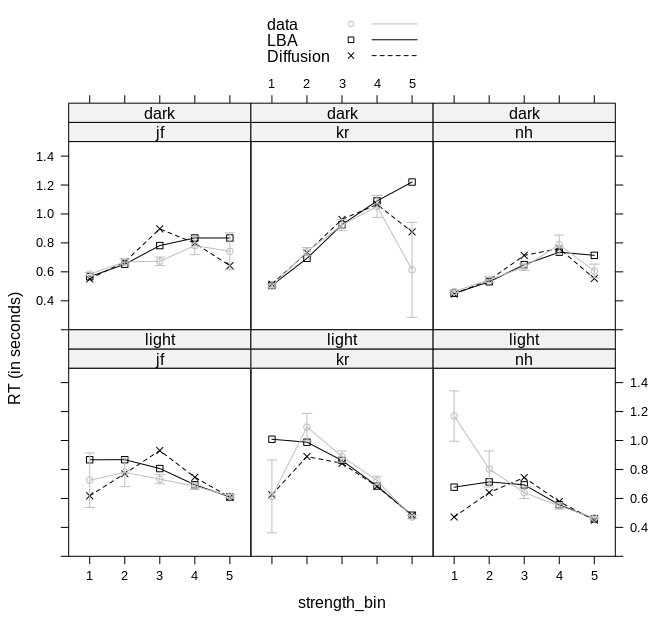Here we see the same observation as for the speed condition. Considerable changes in median RT across strength bins are better captured by the diffusion model than the LBA. This leads to the situation that in most cases the diffusion model can make more accurate prediction for conditions with low numbers of trials.